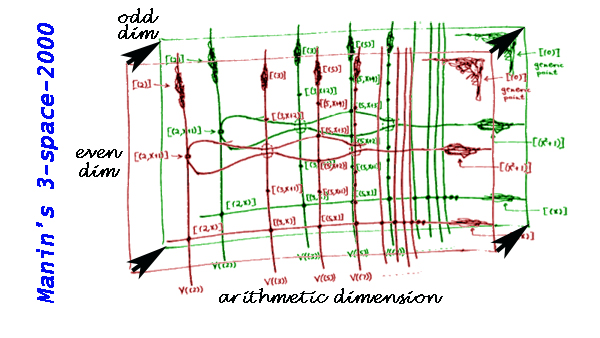Almost three decades ago, Yuri Manin submitted the paper “New dimensions in geometry” to the 25th Arbeitstagung, Bonn 1984. It is published in its proceedings, Springer Lecture Notes in Mathematics 1111, 59-101 and there’s a review of the paper available online in the Bulletin of the AMS written by Daniel Burns.

In the introduction Manin makes some highly speculative but inspiring conjectures. He considers the ring

$$\mathbb{Z}[x_1,\ldots,x_m;\xi_1,\ldots,\xi_n]$$

where $\mathbb{Z}$ are the integers, the $\xi_i$ are the “odd” variables anti-commuting among themselves and commuting with the “even” variables $x_j$. To this ring, Manin wants to associate a geometric object of dimension $1+m+n$ where $1$ refers to the “arithmetic dimension”, $m$ to the ordinary geometric dimensions $(x_1,\ldots,x_m)$ and $n$ to the new “odd dimensions” represented by the coordinates $(\xi_1,\ldots,\xi_n)$. Manin writes :

“Before the advent of ringed spaces in the fifties it would have been difficult to say precisely what me mean when we speak about this geometric object. Nowadays we simply define it as an “affine superscheme”, an object of the category of topological spaces locally ringed by a sheaf of $\mathbb{Z}_2$-graded supercommutative rings.”

Here’s my own image (based on Mumford’s depiction of $\mathsf{Spec}(\mathbb{Z}[x])$) of what Manin calls the three-space-2000, whose plain $x$-axis is supplemented by the set of primes and by the “black arrow”, corresponding to the odd dimension.Manin speculates : “The message of the picture is intended to be the following metaphysics underlying certain recent developments in geometry: all three types of geometric dimensions are on an equal footing”.

Probably, by the addition “2000” Manin meant that by the year 2000 we would as easily switch between these three types of dimensions as we were able to draw arithmetic schemes in the mid-80ties. Quod non.

Twelve years into the new millenium we are only able to decode fragments of this. We know that symmetric algebras and exterior algebras (that is the “even” versus the “odd” dimensions) are related by Koszul duality, and that the precise relationship between the arithmetic axis and the geometric axis is the holy grail of geometry over the field with one element.

For aficionados of $\mathbb{F}_1$ there’s this gem by Manin to contemplate :

“Does there exist a group, mixing the arithmetic dimension with the (even) geometric ones?”

Way back in 1984 Manin conjectured : “There is no such group naively, but a ‘category of representations of this group’ may well exist. There may exist also certain correspondence rings (or their representations) between $\mathsf{Spec}(\mathbb{Z})$ and $x$.”

Published in absolute math noncommutative